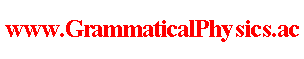since 2006 Help　Sitemap < Forum > < Problems > < Grammatical Unification > < Definition > A coordinate-system is a mapping which maps a mathematical notion on a physical history. A physical law is represented by a mathematical equation. A mathematical equation is a mapping which maps a mathematical notion on 1 or 0. Let M be a coordinate-system and let E represent a physical law. Then the physical law is represented by a mapping E・M-1. M-1 and E・M-1 is defined as follows. [E・M-1](h) ≡ E(M-1(h)) and M(M-1(h)) ≡ h for any physical history h. Nature gives us a physical law as a whole. Who divides it into a coordinate-system and an equation is the human being. There is only physical laws in Nature. Division is made by the human being. So, I assume that we can shift the boundary between the coordinate-system and the equation. --- The idea of the content of this page was published by me in web videos. It had been since 2009/07/17 till 2011/03/08. A video on 2011/03/08 has an expression which reminds us of a tidal wave. It is a part after 10:33 before 11:05 of the video. East Japan Big Earthquake Disaster came on 2011/03/11. Its vibration started at the end of 2011/03/09. Author Yuichi Uda, Write start at 2019/10/06/14:22JST, Last edit at 2019/10/08/14:00JST